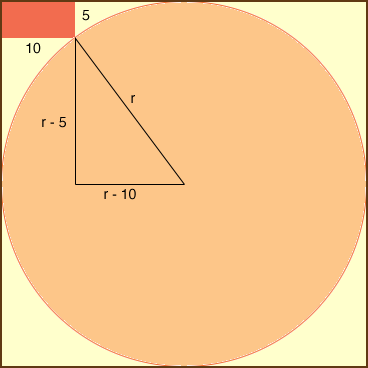SEARCH HOMEMath Central Quandaries & QueriesQuestion from Mercede, a student: there is a circle inside a square... there is a shaded rectangle that measures 5mm X 10mm and they want to know what the radius is. The edge of the shaded rectangle touches a point on the circle.Hi Mercede,

I redrew the diagram you sent and added some lines and dimensions.I let the radius of the circle be r mm and I drew a line segment from the centre of the circle to the point where the rectangle touches the circle. The length of this line segment is r mm. I then constructed a right triangle with this line segment as the hypotenuse. What does Pythagoras' theorem tell you about this triangle?

PennyMath Central is supported by the University of Regina and The Pacific Institute for the Mathematical Sciences.